﻿ 高阶线性矩法在陕北地区洪水频率分析中的应用

# 高阶线性矩法在陕北地区洪水频率分析中的应用Using Higher-Order L-Moments for Flood Frequency Analysis in Northern Shaanxi

Abstract: In order to provide an efficient and reliable theoretical basis for design floods in the northern Shaanxi province, the higher-order L-Moments are applied in flood frequency analysis based on the principles of higher-order L-Moments. The annual maximum flood series of 8 hydrological stations at Jiaokou, Zhangjiashan, Zhaoshiyao, Sudie, Liujia, Zhangcunyi, Linjiacun and Shenmu Rivers are selected for case study. The parameters of Generalized Extreme Value (GEV) distribution and the design floods are estimated. The flood frequency curves are fitted, and the cumulative of squares error is regard as an indicator to evaluate the effect, and compared with the traditional Method of moments. The results show that higher-order L-Moments possess good statistical performance, which can describe the data series much better than lower-order L-Moments in flood analysis. Consequently, this method is reasonable and feasible, and would be provided the basis for the flood quantile calculation.

1. 引言

2. 高阶线性矩

$E\left[{X}_{i,n}\right]=\frac{n!}{\left(i-1\right)!\left(n-i\right)!}{\int }_{0}^{1}x\left(F\right){F}^{i-1}{\left(1-F\right)}^{n-i}\text{d}F$ (1)

${\lambda }_{1}^{\eta }=E\left[{X}_{\left(\eta +1\right)\left(\eta +1\right)}\right]$ (2)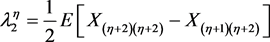(3)

${\lambda }_{3}^{\eta }=\frac{1}{3}E\left[{X}_{\left(\eta +3\right)\left(\eta +3\right)}-2{X}_{\left(\eta +2\right)\left(\eta +3\right)}+{X}_{\left(\eta +1\right)\left(\eta +3\right)}\right]$ (4)

${\lambda }_{4}^{\eta }=\frac{1}{4}E\left[{X}_{\left(\eta +4\right)\left(\eta +4\right)}-3{X}_{\left(\eta +3\right)\left(\eta +4\right)}+3{X}_{\left(\eta +2\right)\left(\eta +4\right)}-{X}_{\left(\eta +1\right)\left(\eta +4\right)}\right]$ (5)

$\eta =0$ 时，高阶线性矩转化为普通线性矩(Hosking, 1990)。随着 $\eta$ 增高，高阶线性矩对随机变量的较大值更为依赖。高阶线性矩的变差系数 ${\tau }_{2}^{\eta }$ ，偏态系数 ${\tau }_{3}^{\eta }$ 和峰态系数 ${\tau }_{4}^{\eta }$ 分别为

${\tau }_{2}^{\eta }=\frac{{\lambda }_{2}^{\eta }}{{\lambda }_{1}^{\eta }}$ (6)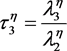(7)

${\tau }_{4}^{\eta }=\frac{{\lambda }_{4}^{\eta }}{{\lambda }_{2}^{\eta }}$ (8)

${\stackrel{^}{\lambda }}_{1}^{\eta }=\frac{1}{{}^{n}C{}_{\eta +1}}\underset{i=1}{\overset{n}{\sum }}{}^{i-1}C{}_{\eta }{x}_{\left(i\right)}$ (9)

${\stackrel{^}{\lambda }}_{2}^{\eta }=\frac{1}{2}\frac{1}{{}^{n}C{}_{\eta +2}}\underset{i=1}{\overset{n}{\sum }}\left({}^{i-1}C{}_{\eta +1}-{}^{i-1}C{}_{\eta }{}^{n-i}C{}_{1}\right){x}_{\left(i\right)}$ (10)

${\stackrel{^}{\lambda }}_{3}^{\eta }=\frac{1}{3}\frac{1}{{}^{n}C{}_{\eta +3}}\underset{i=1}{\overset{n}{\sum }}\left({}^{i-1}C{}_{\eta +2}-2{}^{i-1}C{}_{\eta +1}{}^{n-i}C{}_{1}+{}^{i-1}C{}_{\eta }{}^{n-i}C{}_{2}\right){x}_{\left(i\right)}$ (11)

${\stackrel{^}{\lambda }}_{4}^{\eta }=\frac{1}{4}\frac{1}{{}^{n}C{}_{\eta +4}}\underset{i=1}{\overset{n}{\sum }}\left({}^{i-1}C{}_{\eta +3}-3{}^{i-1}C{}_{\eta +2}{}^{n-i}C{}_{1}+3{}^{i-1}C{}_{\eta +1}{}^{n-i}C{}_{2}-{}^{i-1}C{}_{\eta }{}^{n-i}C{}_{3}\right){x}_{\left(i\right)}$ (12)

${}^{n}C{}_{i}=\frac{n!}{i!\left(n-i\right)!}$ (13)

3. 广义极值分布及其高阶线性矩

$F\left(x\right)=\left\{\begin{array}{l}\mathrm{exp}\left\{-{\left[1-\frac{k}{\alpha }\left(x-\xi \right)\right]}^{\frac{1}{k}}\right\};\text{\hspace{0.17em}}\text{\hspace{0.17em}}\text{\hspace{0.17em}}\text{\hspace{0.17em}}\text{\hspace{0.17em}}\text{\hspace{0.17em}}k\ne 0\\ \mathrm{exp}\left\{-\mathrm{exp}\left[-\frac{1}{\alpha }\left(x-\xi \right)\right]\right\};\text{\hspace{0.17em}}\text{\hspace{0.17em}}\text{\hspace{0.17em}}\text{\hspace{0.17em}}\text{ }k=0\end{array}$ (14)

$x\left(F\right)=\left\{\begin{array}{l}\xi +\frac{\alpha }{k}\left[1-{\left(-\mathrm{ln}F\right)}^{k}\right];\text{\hspace{0.17em}}\text{\hspace{0.17em}}\text{\hspace{0.17em}}k\ne 0\\ \xi -\alpha \mathrm{ln}\left(-\mathrm{ln}F\right);\text{\hspace{0.17em}}\text{\hspace{0.17em}}\text{\hspace{0.17em}}\text{\hspace{0.17em}}\text{\hspace{0.17em}}\text{\hspace{0.17em}}\text{\hspace{0.17em}}\text{\hspace{0.17em}}\text{\hspace{0.17em}}\text{ }k=0\end{array}$ (15)

$k\ne 0$ 时，即为Hosking (1985)  给出的GEV分布概率权重矩(PWM)

${\beta }_{r}={\int }_{0}^{1}x\left(F\right){F}^{r}\text{d}F$ (16)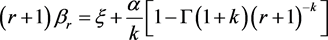(17)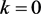时，式(14)为EVI型分布(Gumbel分布)。Greenwood (1979)  给出了GEV分布的PWM

$\left(r+1\right){\beta }_{r}=\xi +\alpha \left[\epsilon +\mathrm{ln}\left(r+1\right)\right]$ (18)

${\lambda }_{1}^{\eta }=\xi +\frac{\alpha }{k}\left[1-\Gamma \left(1+k\right){\left(\eta +1\right)}^{-k}\right]$ (19)

${\lambda }_{2}^{\eta }=\frac{\left(\eta +2\right)\alpha \Gamma \left(1+k\right)}{2!k}\left[-{\left(\eta +2\right)}^{-k}+{\left(\eta +1\right)}^{-k}\right]$ (20)

${\lambda }_{3}^{\eta }=\frac{\left(\eta +3\right)\alpha \Gamma \left(1+k\right)}{3!k}\left[-\left(\eta +4\right){\left(\eta +3\right)}^{-k}+2\left(\eta +3\right){\left(\eta +2\right)}^{-k}-\left(\eta +2\right){\left(\eta +1\right)}^{-k}\right]$ (21)

$\begin{array}{c}{\lambda }_{4}^{\eta }=\frac{\left(\eta +4\right)\alpha \Gamma \left(1+k\right)}{4!k}\left[-\left(\eta +6\right)\left(\eta +5\right){\left(\eta +4\right)}^{-k}+3\left(\eta +5\right)\left(\eta +4\right){\left(\eta +3\right)}^{-k}\\ \text{\hspace{0.17em}}\text{\hspace{0.17em}}-3\left(\eta +4\right)\left(\eta +3\right){\left(\eta +2\right)}^{-k}+\left(\eta +3\right)\left(\eta +2\right){\left(\eta +1\right)}^{-k}\right]\end{array}$ (22)

${\lambda }_{1}^{\eta }=\xi +\alpha \left[\epsilon +\mathrm{ln}\left(\eta +1\right)\right]$ (23)

${\lambda }_{2}^{\eta }=\frac{\left(\eta +2\right)\alpha }{2!}\left[\mathrm{ln}\left(\eta +2\right)-\mathrm{ln}\left(\eta +1\right)\right]$ (24)

${\lambda }_{3}^{\eta }=\frac{\left(\eta +3\right)\alpha }{3!}\left[\left(\eta +4\right)\mathrm{ln}\left(\eta +3\right)-2\left(\eta +3\right)\mathrm{ln}\left(\eta +2\right)+\left(\eta +2\right)\mathrm{ln}\left(\eta +1\right)\right]$ (25)

$\begin{array}{c}{\lambda }_{4}^{\eta }=\frac{\left(\eta +4\right)\alpha }{4!}\left[\left(\eta +6\right)\left(\eta +5\right)\mathrm{ln}\left(\eta +4\right)-3\left(\eta +5\right)\left(\eta +4\right)\mathrm{ln}\left(\eta +3\right)\\ \text{\hspace{0.17em}}\text{\hspace{0.17em}}+3\left(\eta +4\right)\left(\eta +3\right)\mathrm{ln}\left(\eta +2\right)-\left(\eta +3\right)\left(\eta +2\right)\mathrm{ln}\left(\eta +1\right)\right]\end{array}$ (26)

$k={a}_{0}+{a}_{1}\left[{\tau }_{3}^{\eta }\right]+{a}_{2}{\left[{\tau }_{3}^{\eta }\right]}^{2}+{a}_{3}{\left[{\tau }_{3}^{\eta }\right]}^{3}{a}_{4}{\left[{\tau }_{3}^{\eta }\right]}^{4}$ (27)

$\stackrel{^}{\alpha }=\frac{{\lambda }_{2}^{\eta }×\stackrel{^}{k}×2!}{\Gamma \left(1+\stackrel{^}{k}\right)\left(\eta +2\right)\left(-{\left(\eta +2\right)}^{-\stackrel{^}{k}}+{\left(1+\eta \right)}^{-\stackrel{^}{k}}\right)}$ (28)

$\stackrel{^}{\xi }={\stackrel{^}{\lambda }}_{1}^{\eta }-\frac{\stackrel{^}{\alpha }}{\stackrel{^}{k}}\left(1-\Gamma \left(1+\stackrel{^}{k}\right){\left(\eta +1\right)}^{-\stackrel{^}{k}}\right)$ (29)

4. 实例应用

4.1. 绘制频率曲线

$P=\frac{m}{n+1}×100$ (30)Table 1. Coefficients , a 1 , a 2 , a 3 and a 4 for Eq. (27)Table 2. Comparison of quantile errors using different orders L-Moments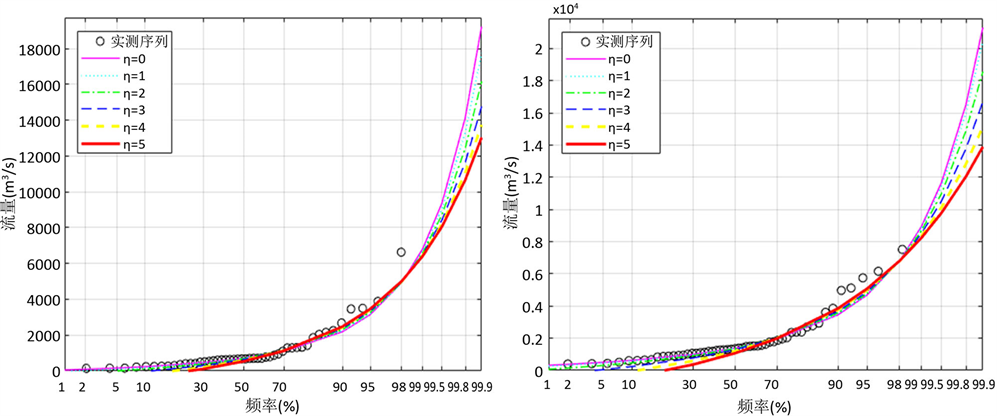(a) 交口河(b) 张家山(c) 赵石窖(d) 绥德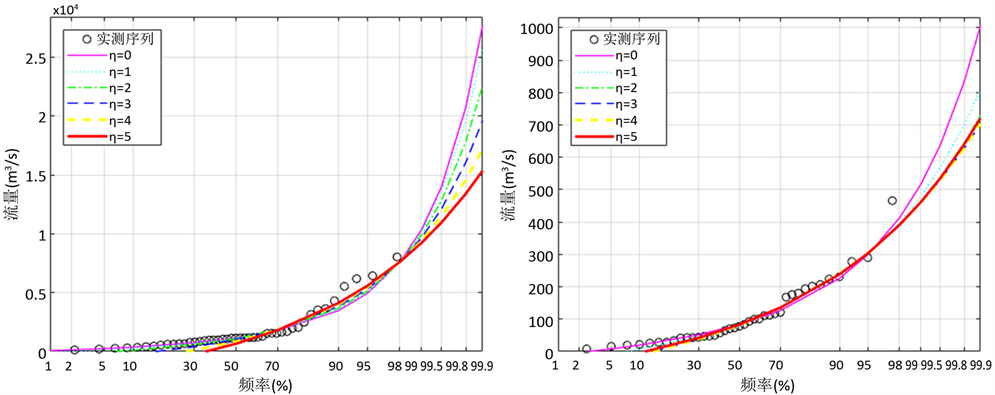(e) 刘家河(f) 张村译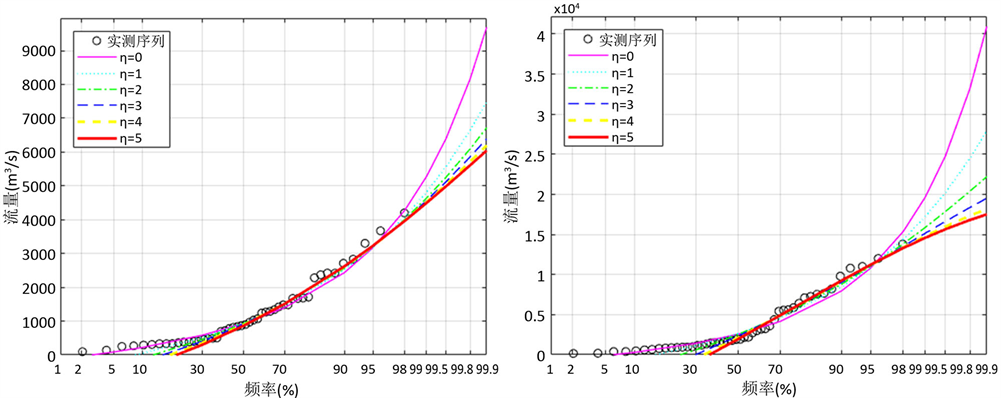(f) 林家村(g) 神木

Figure 1. Fitting of the GEV distribution to annual maximum flows for different orders L-Moments

4.2. 拟合效果分析

$\delta =\underset{i=i|P=50%}{\overset{i|P=98%}{\sum }}{\left(\frac{{x}_{i}-{\stackrel{^}{x}}_{i}}{{x}_{i}}\right)}^{2}$ (31)

5. 结论

 李桃英. 陕西省灾害性洪水类型及成因分析[J]. 水文, 2004, 4(24): 39-42. LI Taoying. The types of disastrous floods in Shaanxi Province and the concerned genetic analysis. Hydrology, 2004, 4(24):39-42. (in Chinese)

 刘攀, 郭生练, 闫宝伟, 李响, 陈璐. 再论分期设计洪水频率与防洪标准的关系[J]. 水力发电学报, 2011, 1(30): 187-192. LIU Pan, GUO Shenglian, YAN Baowei, LI Xiang and CHEN Lu. Supplementary of relationship between seasonal flood frequency and flood control standard. Journal of Hydroelectric Engineering, 2011, 1(30): 187-192.

 王俊珍, 宋松柏. 基于高阶线性矩法的洪水设计值研究[J]. 水力发电学报, 2014,6(33): 33-42. WANG Junzhen, SONG Songbai. Application of higher-order L-Moments for flood quantile estimation. Journal of Hydroelectric Engineering, 2014, 6(33): 33-42. ( in Chinese)

 王俊珍, 宋松柏. 具有历史洪水资料的期望矩法参数估计研究[J]. 水力发电学报, 待刊. WANG Junzhen, SONG Songbai. Expected moments algorithm method for parameters estimation with consideration of historical flood. Journal of Hydroelectric Engineering, in Press. (in Chinese)

 WANG, Q. J. Estimation of the GEV distribution from censored samples by method of partial probability weighted moments. Journal of Hydrology, 1990, (120): 103-114.
https://doi.org/10.1016/0022-1694(90)90144-M

 WANG, Q. J. Using partial probability weighted moments to fit the extreme value distributions to censored samples. Water Resources Research, 1996, 32(6): 1767-1771.

 PANDEY, M. D. Extreme quantile estimation using order statistics with minimum cross-entropy principle. Engineering Mechanics, 2001, (16): 31-42.

 PANDEY, M. D. Minimum cross-entropy method for extreme value estimation using peaks-over-threshold data. Structural Safety, 2001, (23): 345-363.
https://doi.org/10.1016/S0167-4730(02)00008-5

 DENG, J., PANDEY, M. D. Using partial probability weighted moments and partial maximum entropy to estimate quantile from censored samples. Engineering Mechanics, 2009, (24): 407-417.

 DENG, J., PANDEY, M. D. Cross entropy quantile function estimation from censored samples using partial probability weighted moments. Journal of Hydrology, 2008, (363): 18-31.

 詹道江, 徐向阳, 陈元芳. 工程水文学[M]. 北京: 中国水利水电出版社, 2011. ZHAN Daojiang, XU Xiangyang and CHEN Yuanfang. Engineering hydrology. Beijing: China Water Power Press, 2011. (in Chinese)

 董闯, 宋松柏. 群智能优化算法在水文频率曲线适线中的应用[J]. 水文, 2011, 31(2): 20-26. DONG Chuang, SONG Songbai. Application of swarm intelligence optimization algorithm in optimization fitting of hydrologic frequency curve. Journal of China Hydrology, 2011, 31(2): 20-26. (in Chinese)

 谢平, 郑泽权. 水文频率计算有约束加权适线法[J]. 武汉水利电力大学学报, 2000, 33(1): 49-52. XIE Ping, ZHENG Zequan. A constrained and weighted fitting method for hydrologic frequency calculation. Journal of Wuhan University of Hydraulic and Electric Engineering, 2000, 33(1): 49-52. (in Chinese)

 邓育仁, 丁晶, 韦雪艳. 水文计算中的模糊优化适线法[J]. 水电站设计, 1995, 11(4): 43-47. DENG Yuren, DING Jing and WEI Xueyan. Fuzzy optimal curve fitting method in frequency analysis. Design of Hydroelectric Power Station, 1995, 11(4): 43-47. (in Chinese)

 WANG, Q. J. LH moments for statistical analysis of extreme events. Water Resources Research, 1997, 33(12): 2841-2848.
https://doi.org/10.1029/97WR02134

 WANG, Q. J. Using higher probability weighted moments for flood frequency analysis. Journal of Hydrology, 1997, 194(1): 95-106.
https://doi.org/10.1016/S0022-1694(96)03223-4

 HOSKING, J. R. M., WALLIS, J. R. and WOOD, E. F. Estimation of the generalized extreme value distribution by the method of probability weighted moments. Technometrics, 1985, 27(3): 251-261.
https://doi.org/10.1080/00401706.1985.10488049

 GREENWOOD, J. A., LANDWEHR, J. M., MATALAS, N. C., et al. Probability weighted moments: Definition and relation to parameters of several distributions expressible in inverse form. Water Resources Research, 1979, 15(5): 1049-1054.
https://doi.org/10.1029/WR015i005p01049

Top